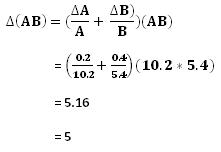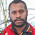Custom Search
If you want to discuss more on this or other issues related to Physics, feel free to leave a message on my Facebook page.

## Monday, October 5, 2009

### How to determine uncertainty in derived quantity when multiplication or division is performed

This is the second part of a series of post on finding uncertainty in derived quantities. You can find the index here and the part on addition and subtraction here.

Now very often when you performed an experiment you meet quantities that need to be processed to obtain a derived quantity such as g, the acceleration due to gravity, or any other derived quantities.

You have already seen the first part on addition and subtraction and now you will see how to do it for multiplication and division.

If in an experiment is performed and the following quantities are measured with their uncertainties.

A = 10.2+-0.2 cm

B = 5.4 +-0.4 cm

Now if you need to process these quantities to find AB and A/B with their uncertainties how would you do it?

Uncertainty in multiplication

How would you determine the value of AB and its uncertainty?

You will have to calculate the value of AB first.

AB = 10.2 *5.4 = 55.o8 =55 (2 sf )

To determine the uncertainty in AB you will have to use the equation below.

Rearranging the equation will give youHence AB = 55+-5 cm2

Remember the uncertainty in A and B are to 1 sf hence the uncertainty in AB must be given to 1 sf.

Uncertainty in division

You are now going to determine the value of A/B and its uncertainty.

You will have to determine the value of A/B first.

A/B = 10.2 /5.4 =1.888 = 1.9

To determine the uncertainty of A/B  you will determine the equation below

Rearranging the equation will give you

Remember the uncertainty in A/B is given to 1 sf since the uncertainties in A and B are given to 1 sf.

Hence A/B = 1.9 +-0.2

With these two formula you can thus determine the uncertainty in any derived quantities that involves multiplication and division.

Now you can move to the next part where the method to determine uncertainty of derived quantities where powers,  square root, etc are involved.

1.Thank You for your clear instructions, i was able to follow & comprehend thoroughly. Very much appreciated

2.Thanks for the help!

3.THANK YOU SO MUCH!

4.Thank You, your help/instructions were of great help and are greatly appreciated

5.but i want to know how do you get 0.2 as the uncertainty

6.i really appreciate for your instructions thanky you so much

7.so clear! it was really helpful

8.thanks for your help but i wanted to know how to calculate using physical quantities

9.Thanks so much for your clear and understandable way of explaining calculating numerical values involved with uncertainty. So much joy.

10.Once again thanks so much